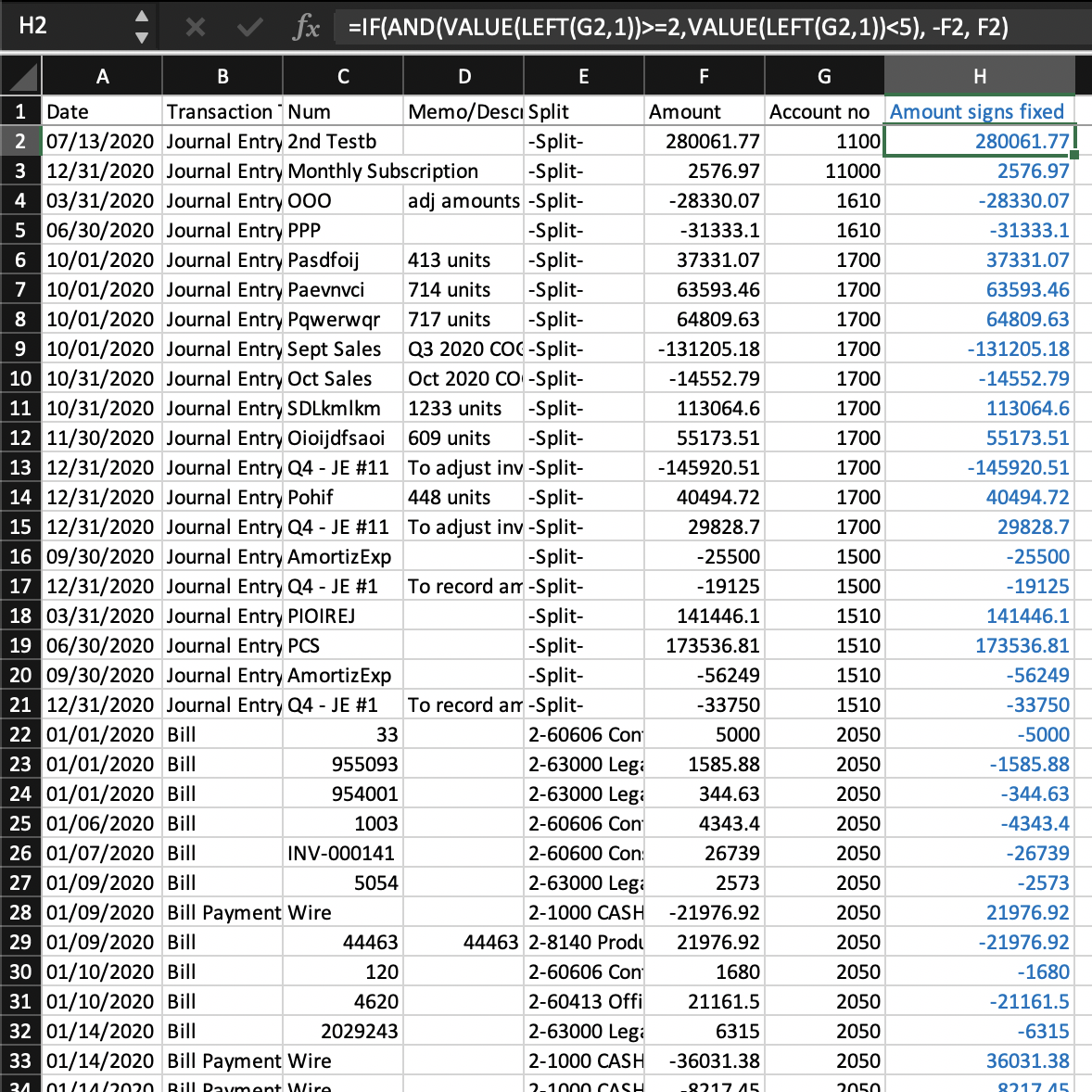# Data Formatting Guide: Flipping signs for a range of accounts or based on condition(s)

• Updated

## Summary

Within this article, you will find data formatting tips and tricks for flipping signs for a range of accounts or based on condition(s). This will help you format your data for use within MindBridge.

## Example: Flipping signs for a range of accounts using formulas

Some general ledgers come with an Amount column, but with each account having a default positive balance. Recall that if Amount is used, instead of separate Debit and Credit columns, all debits must be positive and credits negative. To fix the signs for such a GL, you can filter on the account numbers that should have their signs reversed and reverse just those, or you can use formulas to do so.

Here is an example of how to use formulas. Suppose in this GL, all account numbers in the 2000s through 4000s are liability or equity accounts that should have default credit balances, but all accounts have default positive amounts. Thus the sign on the Amount need to be flipped for any account number beginning with 2 through 4.

We create a new "Amount signs fixed" column at the end, in column H (in this example), with this formula in cell H2:

`=IF(AND(VALUE(LEFT(G2,1))>=2,VALUE(LEFT(G2,1))<5), -F2, F2)`To explain what the formula is doing:

• LEFT(G2,1) takes the first character in cell G2 (in this case, 1)
• Wrapping it around VALUE() turns it into a number so that we can compare values (string operations such as LEFT(), RIGHT(), and MID() return string instead of number types)
• The AND() function says that if the first character in G2 is greater than or equal to 2 and less than 5 (that is, both conditions are true)
• The outer function is IF(). If the AND() function is true (i.e., the first character in G2 is greater than or equal to 2 and less than 5), then return -F2 (i.e., flip the sign on the Amount), otherwise return F2 (just return the amount without flipping the sign)

Ensure that the balance in the "Amount signs fixed" net out to zero.

## Example: Flipping signs based on debit/credit indicator (SAP)

Sometimes there will be a column indicating whether the amount is a debit or a credit, such as in the following SAP extract where H indicates credits and S indicates debits.

We create a new "Amount signs fixed" column at the end, in column D (in this example), with this formula in cell D2:

`=IF(B2="H",-C2,C2)`Anything else on your mind? Chat with us or submit a request for further assistance.